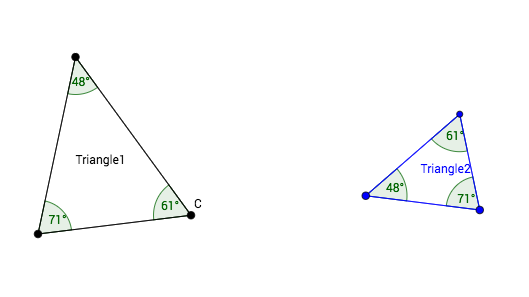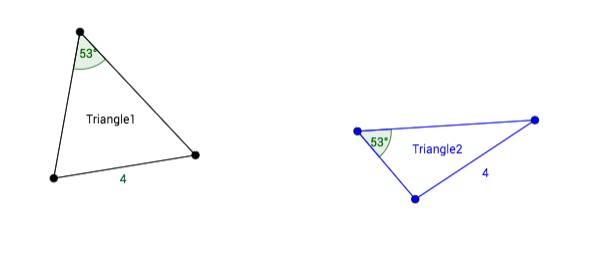# Triangle Congruence Criteria = "Shortcuts"?

## Do we really need to know ALL the corresponding parts are congruent?

Sometimes we may know some of the corresponding parts of two triangles are congruent, but not all 6 pairs of corresponding parts. When do we have enough information to conclude the triangles must be congruent, and when is there room for doubt? We will look for criteria which allow us to conclude two triangles are congruent without actually showing/knowing all 6 pairs of corresponding parts are congruent.

## ONE pair of congruent corresponding parts is not enough!

To convince yourself: 1) Draw a pair of NON-congruent triangles which have one pair of congruent sides. 2) Draw a pair of NON-congruent triangles which have one pair of congruent angles.

## TWO pairs of congruent corresponding parts is still not enough!

There are 4 cases: SS, AA(A), SA adjacent, and SA opposite....AA(A) is not enough! Two pairs of congruent angles actually implies three pairs of congruent angles. Do you know why? In any case, Triangle1 and Triangle2 are clearly not congruent.SA opposite is not enough! When the congruent pair of angles is across from the congruent pair of sides, again, there can be non-congruent triangles.
Come up with your own examples of pairs of non-congruent triangles for the SS and SA adjacent cases.

## Up Next...

Since SS is not enough, we will next consider adding one more pair of corresponding parts. The possibilities are SSS (add congruent pair of sides), SAS (add congruent included pair of angles), and SSA (add congruent non-included pair of angle).